# resid

Compute and test residuals

## Syntax

``resid(Data,sys)``
``resid(Data,sys,Linespec)``
``resid(Data,sys1,...,sysn)``
``resid(Data,sys1,Linespec1,...,sysn,Linespecn)``
``resid(___,Options)``
``resid(___,Type)``
``````[E,R] = resid(Data,sys)``````

## Description

example

````resid(Data,sys)` computes the 1-step-ahead prediction errors (residuals) for an identified model, `sys`, and plots residual-input dynamics as one of the following, depending on the data in`Data`:For time-domain data, `resid` plots the autocorrelation of the residuals and the cross-correlation of the residuals with the input signals. The correlations are generated for lags -25 to 25. To specify a different maximum lag value, use `residOptions`. The 99% confidence region marking statistically insignificant correlations displays as a shaded region around the X-axis. For frequency-domain data, `resid` plots a bode plot of the frequency response from the input signals to the residuals. The 99% confidence region marking statistically insignificant response is shown as a region around the X-axis.To change display options, right-click the plot to access the context menu. For more details about the menu, see Tips.```

example

````resid(Data,sys,Linespec)` sets the line style, marker symbol, and color.```

example

````resid(Data,sys1,...,sysn)` computes and plots the residual of multiple identified models `sys1`,...,`sysn`.```

example

````resid(Data,sys1,Linespec1,...,sysn,Linespecn)` sets the line style, marker symbol, and color for each system.```

example

````resid(___,Options)` specifies additional residual calculation options. Use `Options` with any of the previous syntaxes.```

example

````resid(___,Type)` specifies the plot type. Use `Type` with any of the previous syntaxes.```

example

``````[E,R] = resid(Data,sys)``` returns the calculated residuals, `E`, and residual correlations, `R`. No plot is generated.```

## Examples

collapse all

```load iddata1 data = z1;```

Estimate an ARX model.

`sys = arx(data,[1 1 0]);`

Plot the autocorrelation of the residuals and cross-correlation between the residuals and the inputs.

`resid(data,sys)`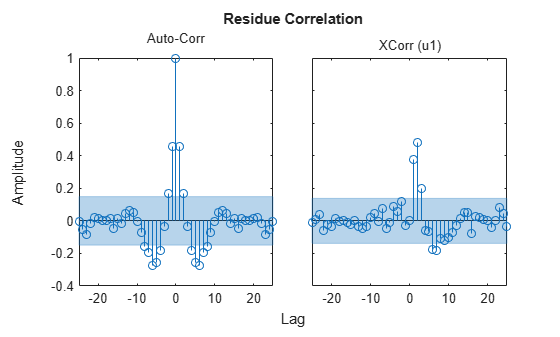The correlations are calculated until the default maximum lag, 25. The 99% confidence region marking statistically insignificant correlations displays as a shaded region around the X-axis.

Convert data to frequency domain.

`data2 = fft(data);`

Compute the residuals for identified model, `sys`, and the frequency-domain data. Plot the residual response using red crosses.

`resid(data2,sys,'rx')`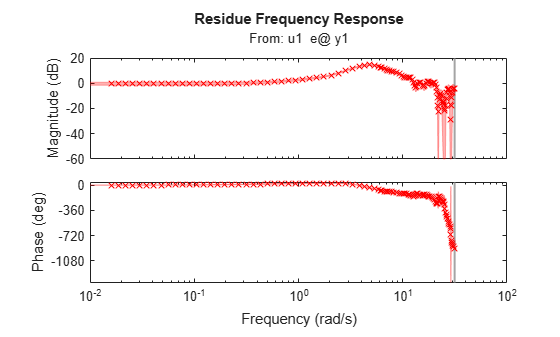For frequency-domain data, `resid` plots the Bode plot showing frequency response from the input to the residuals.

`load iddata1`

Estimate an ARX model.

`sys1 = arx(z1,[1 1 0]);`

Estimate a transfer function model.

`sys2 = tfest(z1,2);`

Plot the correlations of the residuals.

`resid(z1,sys1,'b',sys2,'r')`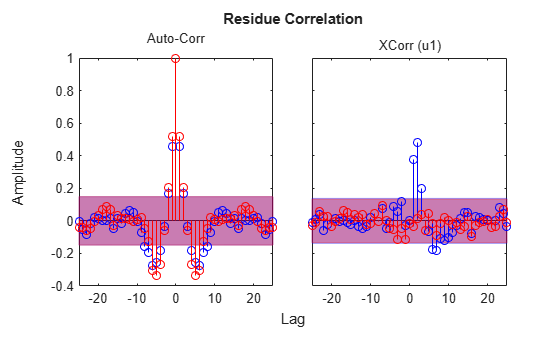The cross-correlation between residuals of `sys2` and the inputs lie in the 99% confidence band for all lags.

`load iddata1`

Estimate an ARX model.

`sys = arx(z1,[1 1 0]);`

Specify the maximum lag for residual correlation calculations.

`opt = residOptions('MaxLag',35);`

Plot the impulse response from the input to the residuals.

`resid(z1,sys,opt,'ir')`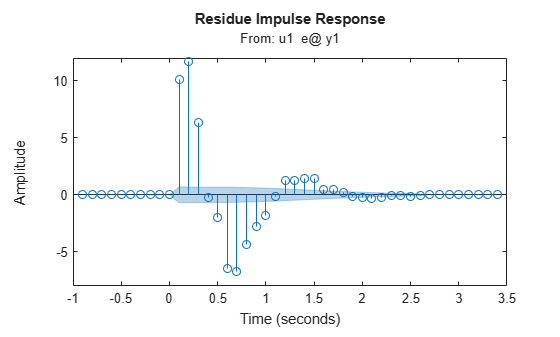`load iddata7`

The data is a two-input, single-output dataset.

Estimate an ARX model.

`sys = tfest(z7,2);`

Calculate the residuals and their autocorrelations and cross-correlations with inputs.

`[E,R] = resid(z7,sys);`

`R` is a 26-by-3-by-3 matrix of correlations. For example,

• `R(:,1,1)` is the autocorrelation of the residuals until lag 25.

• `R(:,1,2)` is the cross-correlation of the residuals with the first input,until lag 25.

`E` is an iddata object with the residuals as output data and the inputs of validation data (z7) as input data. You can use `E` to identify error models and analyze the error dynamics.

Plot the error.

`plot(E)`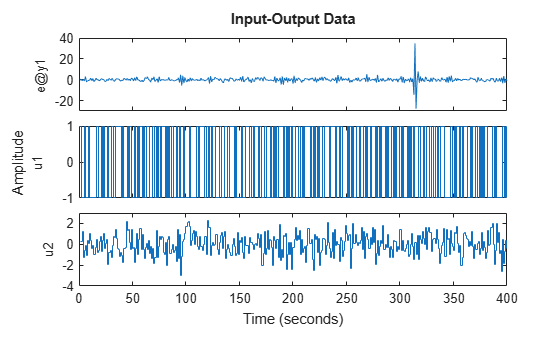Estimate impulse response between inputs and residuals. Plot them with a 3 standard deviation confidence region.

```I = impulseest(E); showConfidence(impulseplot(I,20),3)```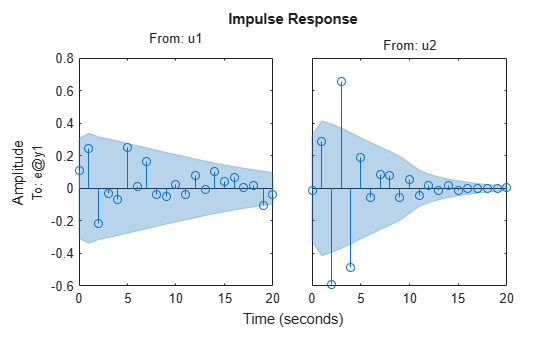## Input Arguments

collapse all

Validation input-output data, specified as an `iddata` object. `Data` can have multiple input-output channels. When `sys` is linear, `Data` is time-domain or frequency-domain. When `sys` is nonlinear, `Data` is time-domain.

System used for computing residuals, specified as an identified linear or nonlinear model.

Example: `idpoly`

Line style, marker symbol, and color, specified as a character vector. For more information, see `plot`. When `Type` is specified as `'corr'`, only the line style is used.

Example: `'Linespec','kx'`

Residual analysis options, specified as an `residOptions` option set.

Plot type, specified as one of the following values:

• `'corr'` — Plots the autocorrelation of the residuals, `e`, and the cross-correlation of the residuals with the input signals, `u`. The correlations are generated for lags -25 to 25. Use `residOptions` to specify a different maximum lag value. The 99% confidence region marking statistically insignificant correlations is also shown as a shaded region around the X-axis. The computation of the confidence region is done assuming `e` to be white and independent of `u`.

`'corr'` is default for time-domain data. This plot type is not available for frequency-domain data.

• `'ir'` — Plots the impulse response up to lag 25 of a system from the input to the residuals. The `impulseest` command first estimates the impulse response model with `e` as output data and `u` as inputs. Then `impulseest` calculates the impulse response of the estimated model. The 99% confidence region marking statistically insignificant response displays as a shaded region. A low magnitude indicates a reliable model.

This plot type is not available for frequency-domain data.

• `'fr'` — The frequency response from the input to the residuals (based on a high-order FIR model) is shown as a Bode plot. The 99% confidence region marking statistically insignificant response displays as a shaded region. A low magnitude in the frequency range of interest indicates a reliable model.

`'fr'` is default for frequency-domain data.

## Output Arguments

collapse all

Model residuals, returned as an `iddata` object. The residuals are stored in `E.OutputData`, and the inputs are stored in `E.InputData`. Use `E` to build models that describe the dynamics from the inputs to the residuals. The dynamics are negligible if `sys` is a reliable identified model.

Correlations of the residuals, returned as one of the following:

• Matrix of doubles — For time-domain-data

`R` is a matrix of size M+1-by-(ny+nu)-by-(ny+nu). Where, M is the maximum lag specified in `Options`, ny is the number of outputs, and nu is the number of inputs. The default value of M is 25.

At each lag k (`k = 0:M`), `R(k,i,j)` is the expectation value, `<Z(t,i).Z(t+k-1,j)>`. Here, `Z = [E.OutputData,E.InputData]`.

For example, for a two-output, single-input model, ```Z = [e1,e2,u1]```. Where, e1 is the residual of the first output, e2 is the residual of the second output, and u1 is the input. `R` is a `26`-by-`3`-by-`3` matrix, where:

• `R(5,1,2) = <e1(t).e2(t+4)>` is the cross-correlation at lag 4 between e1 and e2.

• `R(5,1,3) = <e1(t).u1(t+4)>` is the cross-correlation at lag 4 between e1 and u1.

• `R(5,1,1)`, `R(5,2,2)`, `R(5,3,3)` are the autocorrelations at lag 4 for e1, e2, and u1, respectively.

• `[]` — For frequency-domain data

## Tips

• Right-clicking the plot opens the context menu, where you can access the following options:

• Systems — Select systems to view the residual correlation or response plots. By default, all systems are plotted.

• Show Confidence Region — View the 99% confidence region marking statistically insignificant correlations. Applicable only for the correlation plots.

• Data Experiment — For multi-experiment data only. Toggle between data from different experiments.

• Characteristics — View data characteristics. Not applicable for correlation plots.

• Peak Response — View peak response of the data.

• Confidence Region — View the 99% confidence region marking statistically insignificant response.

• Show — Applicable only for frequency-response plots.

• Magnitude — View magnitude of frequency response.

• Phase — View phase of frequency response.

• I/O Grouping — For datasets containing more than one input or output channel. Select grouping of input and output channels on the plot. Not applicable for correlation plots.

• None — Plot input-output channels in their own separate axes.

• All — Group all input channels together and all output channels together.

• I/O Selector — For datasets containing more than one input or output channel. Select a subset of the input and output channels to plot. By default, all output channels are plotted.

• Grid — Add grids to the plot.

• Normalize — Normalize the y-scale of all data in the plot. Not applicable for frequency-response data.

• Full View — Return to full view. By default, the plot is scaled to full view.

• Initial Condition — Specify handling of initial conditions.

Specify as one of the following:

• Estimate — Treat the initial conditions as estimation parameters.

• Zero — Set all initial conditions to zero.

• Absorb delays and estimate — Absorb nonzero delays into the model coefficients and treat the initial conditions as estimation parameters. Use this option for discrete-time models only.

• Properties — Open the Property Editor dialog box to customize plot attributes.

 Ljung, L. System Identification: Theory for the User. Upper Saddle River, NJ: Prentice-Hall PTR, 1999, Section 16.6.

## Support